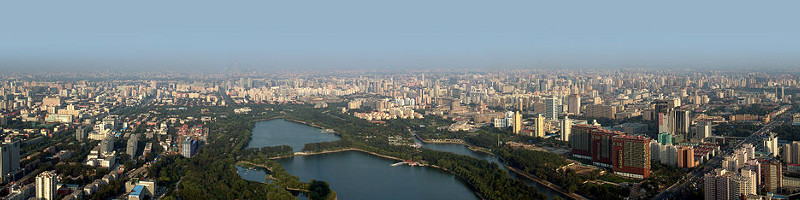### Redundant Inertial Sensor Demonstration

 example_MultiINSvsSRIMU.m A strictly theoretical example comparing multi-ins and single-INS approaches for SRIMU based systems. example_OptimalFusion.m An example showing the optimal fusion of inertial sensors for skew redundant inertial measurement units example_SRIMUNav.m An example showing the different methods of processing SRIMU data in a navigation system

The following function are just simple helpers used in the above scripts

 cp_M_v000.m cp_redsys_v000.m Reduced order system model for redundant yet identical sensors. cp_T_v000.m st_size_v000.m